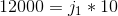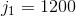## Example Questions

### Example Question #1 : How To Use The Inverse Variation Formulavaries inversely with. When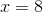,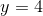. What doesequal when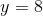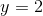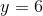Explanation:

1. Use the given values ofandto solve for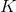: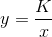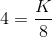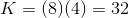2. Solve forwhenin the above equation: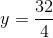### Example Question #2 : How To Use The Inverse Variation Formula

If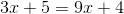, what is the value of?Explanation:

To solve this algebraic equation, subtractfrom both sides, and then subtractfrom both sides.

We end up with the equation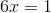, for which the solution is: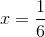### Example Question #3 : How To Use The Inverse Variation Formula

In a given set of experiments, the values of two variables are always inversely proportional. If in the first experiment the first variable wasand the second was, what could you expect the second variable to be if the first is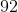in a later experiment?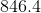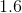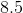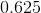Explanation:

Recall that inverse variation means that when one variable increases, the other decreases. This gives you the following equation: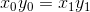Now, for our data, we know: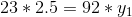You merely have to solve for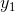: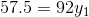Divide by: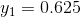### Example Question #4 : How To Use The Inverse Variation Formula

Throughout a party, the number of joyful non-philosophers in a room is always inversely proportional to the number of philosophers in the room. The room begins with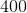people,of whom are philosophers. Later in the day, there arephilosophers in the room. How many joyful non-philosophers are at the party at the later time?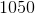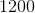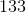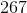Explanation:

Recall that inverse variation means that when one variable increases, the other decreases. This gives you the following equation: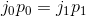For our data, this means: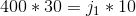You merely need to solve for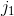: